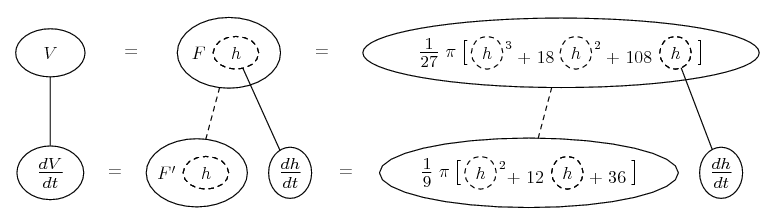# Thread: Rate of Change in a Truncate Cone?

1. ##Rate of Change in a Truncate Cone?

There is a truncate cone shaped mug and you are pouring coffee with 20 cm^3/s. The upper radius is 4 and the lower one is 2. What is the rate of change in height when it's half full?

I wrote V=pi. h/3 (r^2-4) but I don't know dr/dt? How do I solve it?

2.Originally Posted by twipotThere is a truncate cone shaped mug and you are pouring coffee with 20 cm^3/s. The upper radius is 4 and the lower one is 2. What is the rate of change in height when it's half full?

I wrote V=pi. h/3 (r^2-4) but I don't know dr/dt? How do I solve it?
Use the cone volume formula, then differentiate with the chain rule...

Just in case a picture helps...... where

$\displaystyle V = F(h)$

$\displaystyle = \frac{1}{3} \pi R^2 (h + 6) - \frac{1}{3} \pi (2)^2 (6)$

$\displaystyle = \frac{1}{3} \pi (2 + \frac{1}{3}h)^2 (h + 6) - \frac{1}{3} \pi (2)^2 (6)$

$\displaystyle = \frac{1}{3} \pi [(2 + \frac{1}{3}h)^2 (h + 6) - (2)^2 (6)]$

$\displaystyle = \frac{1}{3} \pi [(4 + \frac{1}{9}h^2 + \frac{4}{3}h) (h + 6) - 24]$

$\displaystyle = \frac{1}{3} \pi [4h + \frac{1}{9}h^3 + \frac{4}{3}h^2 + 24 + \frac{2}{3}h^2 + \frac{24}{3}h - 24]$

$\displaystyle = \frac{1}{3} \pi [\frac{1}{9}h^3 + 2h^2 + 12h]$

$\displaystyle = \frac{1}{27} \pi [h^3 + 18h^2 + 108h]$

So

$\displaystyle F'(h) = \frac{dV}{dh}$

$\displaystyle = \frac{1}{27} \pi [3h^2 + 36h + 108]$

So, $\displaystyle \frac{dh}{dt}$

$\displaystyle = \frac{\frac{dV}{dt}}{\frac{1}{27} \pi [3h^2 + 36h + 108]}$

$\displaystyle = \frac{20}{\frac{1}{27} \pi [3(3)^2 + 36(3) + 108]}$

$\displaystyle = \frac{20 * 27}{\pi [3(3)^2 + 36(3) + 108]}$

$\displaystyle = \frac{540}{\pi (27 + 108 + 108)}$

$\displaystyle = \frac{540}{243 \pi}$

$\displaystyle = \frac{20}{9 \pi}$

(cm/s).... is the chain rule. Straight continuous lines differentiate downwards (integrate up) with respect to x, and the straight dashed line similarly but with respect to the dashed balloon expression (the inner function of the composite which is subject to the chain rule).

Sorry you never got a picture of the cup itself, which is what you were probably hoping for! But the most important thing with related rates is usually the chain rule.

__________________________________________

Don't integrate - balloontegrate!

http://www.ballooncalculus.org/examples/gallery.html

http://www.ballooncalculus.org/asy/doc.html

3. Can you help further with the solution? I'm not any good at solving rate of change problems... By the way, the height is 6 cm.

4. Thanks for hanging on - pic in a mo. Get the volume in terms of h by getting r in terms of h. Sketch the cross-section so you can see that r at the surface of the coffee is 2 plus another third of h the depth (which is max 6). So get the volume from that, and from subtracting the non-existant part of the cone...

change, cone, rate, truncate